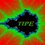Hi everybody!

I'm Jorge Tipe from Peru, I'm one of the coaches of the Peruvian mathematical team. In Lima, we meet once or twice a week to have lessons and solve some problems. Our group consists of about twelve students, they will represent my country in the different mathematical olympiads such as Cono Sur Mathematical Olympiad, Iberoamerican, Rioplatense and of course IMO. Later I will comment about these olympiads, probably you never heard about some of them.

The idea of #PeruMOTraining is to share with you some material such as

• Lessons with solved problems and some additional problems for you. These lessons will be divided in parts I, II, III,... as needed.

• Problems or set of problems for training. I will try to use some not well known problems including those of the Peruvian Mathematical Olympiad and the olympiads above mentioned.

Starting for now, I will have new material for you every week!

Jorge.Note by Jorge Tipe
7 years, 4 months ago

This discussion board is a place to discuss our Daily Challenges and the math and science related to those challenges. Explanations are more than just a solution — they should explain the steps and thinking strategies that you used to obtain the solution. Comments should further the discussion of math and science.

When posting on Brilliant:

• Use the emojis to react to an explanation, whether you're congratulating a job well done , or just really confused .
• Ask specific questions about the challenge or the steps in somebody's explanation. Well-posed questions can add a lot to the discussion, but posting "I don't understand!" doesn't help anyone.
• Try to contribute something new to the discussion, whether it is an extension, generalization or other idea related to the challenge.

MarkdownAppears as
*italics* or _italics_ italics
**bold** or __bold__ bold
- bulleted- list
• bulleted
• list
1. numbered2. list
1. numbered
2. list
Note: you must add a full line of space before and after lists for them to show up correctly
paragraph 1paragraph 2

paragraph 1

paragraph 2

[example link](https://brilliant.org)example link
> This is a quote
This is a quote
    # I indented these lines
# 4 spaces, and now they show
# up as a code block.

print "hello world"
# I indented these lines
# 4 spaces, and now they show
# up as a code block.

print "hello world"
MathAppears as
Remember to wrap math in $$ ... $$ or $ ... $ to ensure proper formatting.
2 \times 3 $2 \times 3$
2^{34} $2^{34}$
a_{i-1} $a_{i-1}$
\frac{2}{3} $\frac{2}{3}$
\sqrt{2} $\sqrt{2}$
\sum_{i=1}^3 $\sum_{i=1}^3$
\sin \theta $\sin \theta$
\boxed{123} $\boxed{123}$

Sort by:

Aw. Thank you very much sir. I'm so glad that you'll be training us with other teachers too :)

- 7 years, 4 months ago

I can't wait! Here is the link to the first problem! It's a geometry proof :)

Staff - 7 years, 4 months ago

hi Jorge could you please solve the problem which I reshared a couple of hours ago

- 7 years, 3 months ago

What problem?

- 7 years, 3 months ago

minimum value of z such that z^3 =a^4 +b^4+(a+b)^4 for distinct positive integers a and b sorry for not making that clear Jorge would really love to see a solution to that problem looking forward to hearing from you Des O Carroll

- 7 years, 3 months ago

The first step is to factorize $a^4+b^4+(a+b)^4=2(a^2+ab+b^2)^2$. Hope this helps!

- 7 years, 3 months ago

thanks Jorge but I got that far then I considered (a^2 +ab +b^2)^2 =2^8 ; 2^14;2^21etc but got no solution then I considered (a^2 +ab+b^2)^2=2^2.3^6;2^2.3^12 etc at this stage all I want is the answer can you give it to me Thanks Jorge

- 7 years, 3 months ago